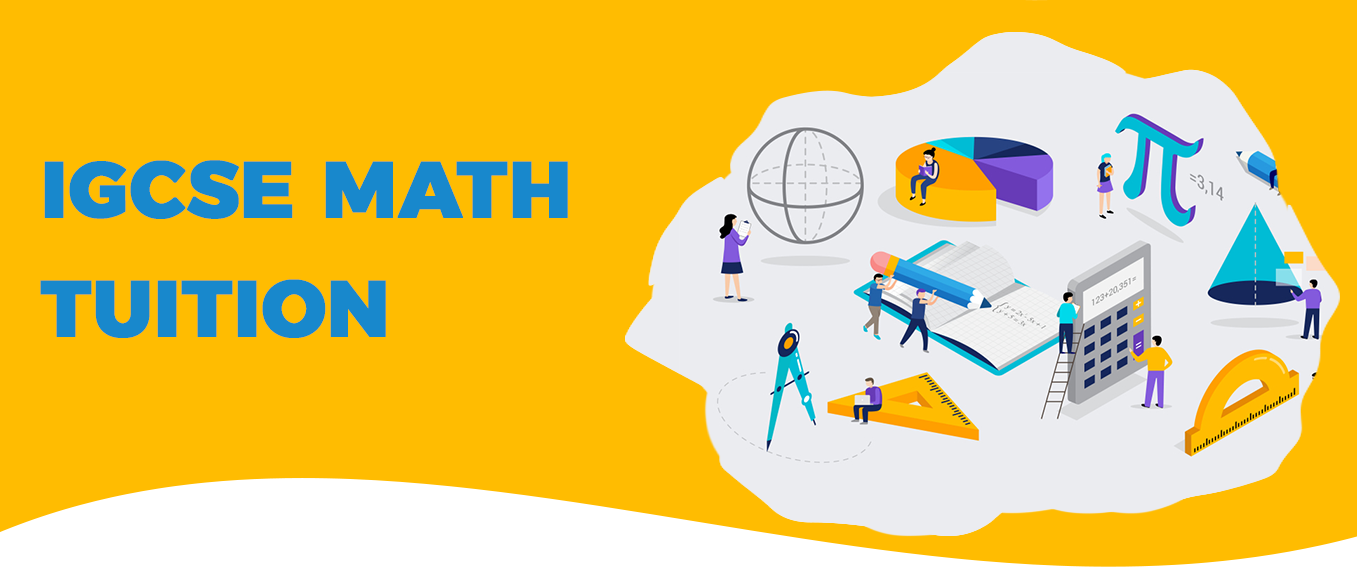# Igcse Maths TuitionBaccalaureate Classes provides well qualified and experienced IGCSE maths tutors in India. As we know that Cambridge educational programmes are some of the most popular and widely accepted educational curriculum in the world. The Cambridge IGCSE curriculum provides a wide range of course for the primary, lower secondary, higher secondary and pre university level students. The IGCSE mathematics course offered by Cambridge has many variants. We have well trained team of IGCSE math tutor for each of the variant. These mathematics courses offered by Cambridge Assessment International Education are Mathematics - 580, Mathematics – Additional - 0606, Mathematics – Additional (US) - 0459, Mathematics – international - 0607, Mathematics (9-1) - 0980 and Mathematics (US) - 0444. The IGCSE Maths (0580) has two variants which are core and extended. The extended curriculum consists of core curriculum as well as few supplementary topics and the level of examination papers for both the courses differ a lot. The grades available for core curriculum is C D E F G whereas grades available for the extended curriculum are A* A B C D E. Our online IGCSE maths tutor teach core and extended students in separate batches. Students are allotted the core and extended maths as per their abilities and previous performance. The main syllabus of the course has four major sections which are Number, Algebra, Shape and space, Probability and statistics. Algebra section contains basic algebra, graphs and coordinates geometry. The shape and space section contains geometry, mensuration, trigonometry, vectors and transformations. The distribution of marks for different sections is different for core and extended courses. Our team of IGCSE maths tutor in Delhi and Noida has developed the topic wise worksheets for every section. For the IGCSE core mathematics, students are supposed to write paper 1 and paper 3. The duration of Paper 1 and paper 3 is one hour and two hours respectively. Paper 1 weighs 35% of the total grade and paper 3 weighs 65% of the total grade. For IGCSE extended mathematics, students are supposed to write paper 2 and paper 4. The duration of paper 2 and paper 4 is one hour thirty minutes and two hours thirty minutes respectively. Paper 2 weighs 23% of the total grade and paper 4 weighs 65% of the total grade. Due to the complexity of the extended course, many students feel the need of IGCSE maths tuition to understand the topic efficiently.

Many students opt for the Cambridge IGCSE International mathematics due to their keen interest to learn the subject. The main aim of the course is to make the students more confident and competent in IGCSE mathematics course. Our team of IGCSE maths home tutor is very well trained in teaching this advanced course. IGCSE international maths has also two variants which are core and extended. The main topics for this course are number, algebra, functions, coordinate geometry, geometry, vectors and transformations, mensuration, trigonometry, sets, probability and statistics. A graphic display calculator is needed for this course. The IGCSE international core maths students are supposed to write paper 1, paper 3 and paper 5. The duration of these there papers is forty five minutes, one hour forty five minutes and one hour ten minutes respectively. Paper 1 , 3 and 5 weighs 25%, 60% and 15% of the final grade respectively. Our every IGCSE maths home tutor in Gurgaon conducts the topic wise assessment at student’s home after completion of each topic. The IGCSE international extended maths students are supposed to write paper 2, paper 4 and paper 6. The duration of these three papers is forty five minutes, two hours fifteen minutes and one hour forty minutes respectively. Paper 2, 4 and 6 weighs 20%, 60% and 20% of the total grade respectively. Our group of IGCSE maths home tutor in Delhi and Noida has developed topic wise revision notes for al the topics of this course. The use of graphic display calculator makes the IGCSE international maths paper more innovative as well as interesting for the students.